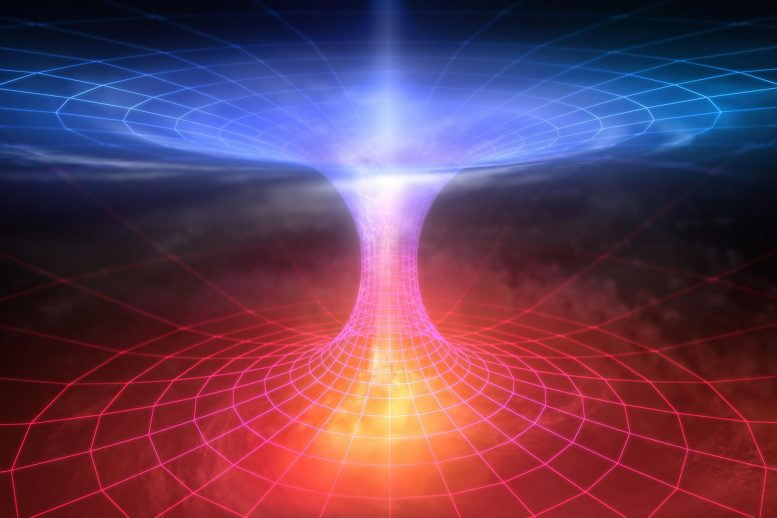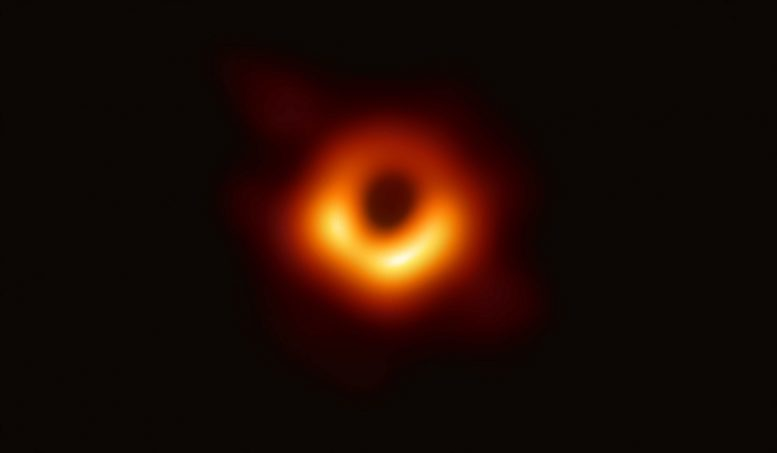# A Quantum Physics Approach to a Singularity ProblemOne of the major issues in general relativity that separates it from other descriptions of the universe, like quantum physics, is the existence of singularities. Singularities are points that when mathematically described give an infinite value and suggest areas of the universe where the laws of physics would cease to exist — i.e. points at the beginning of the universe and at the center of black holes.

A new paper in Nuclear Physics B, published by Roberto Casadio, Alexander Kamenshchik, and Iberê Kuntz from the Dipartimento di Fisica e Astronomia, Università di Bologna, Italy, suggests that extending the treatment of singularities in classical physics into quantum physics could help to solve this disparity between branches of physics.

“No description of nature is perfect and complete. Every theory has its domain of applicability, beyond which it breaks down and its predictions no longer make sense,” Casadio says. As an example, he cites Newton’s theories, which are still robust enough to send rockets to space, but fall down when describing the very small, or the tremendously massive.A new quantum approach to the problem of singularities could answer the question of what happens at the center of a black hole like this one found in the galaxy M87. Credit: EHT

“This is a serious issue because general relativity — the theory that best describes the gravitational interaction at present — predicts the existence of singularities quite generically,” Casadio says. “It is like having a hole in space, where nothing can exist, but into which observers and everything else will fall nonetheless.”

Casadio suggests that this can be envisaged as a piece of paper with a small hole in it. “You can move the tip of your pen on the paper, which represents the movement of a particle, but if you reach the hole your pen suddenly stops drawing and the particles suddenly disappear,” he says. “This illustrates how singularities are theoretical obstacles preventing us from fully understanding nature.”

Casadio adds that the fact that physics ceases to exist at singularities leads to unanswered questions such as: What really happened at the beginning of the universe? Was everything born out of a point that never really existed? What happens to a particle when it falls into the center of a black hole?

“These open questions are the very reason we are compelled by our curiosity to pursue this line of investigation,” he says. “Our approach heavily relies on the methods of Quantum field theory (QFT): the framework that combines quantum mechanics and special relativity and gives rise to the very successful standard model of particle physics.”

The authors used the tools of QFT to construct a mathematical object that can signal the presence of singularities in experimentally measurable quantities. This object, which they have named the “functional winding number” is non-zero in the presence of singularities and vanishes in their absence.

This approach has revealed that certain singularities predicted theoretically do not affect quantities that can in principle be measured experimentally, and therefore remain harmless mathematical constructs.

“If our formalism survived scientific scrutiny and turned out to be the correct approach, it would suggest the existence of a very deep physical principle, so the choices of physical variables are rather unimportant,” Casadio concludes. “This could be consequential for our understanding of physics, even beyond the subject of singularities.”

Reference: “Covariant singularities in quantum field theory and quantum gravity” by Roberto Casadio, Alexander Kamenshchik and Iberê Kuntz, 26 July 2021, Nuclear Physics B.
DOI: 10.1016/j.nuclphysb.2021.115496

#### 5 Commentson "A Quantum Physics Approach to a Singularity Problem"

1.Christopher Lombness | February 17, 2022 at 2:15 pm | Reply

I have an expanding theory of correlation in the particle physics of General Relativity to Direct Current/DC electrical formula with Mass being the parallel to Resistance and the Constant being parallel to Current. The energy is of course parallel to Electrical Power.

e=mc²
P=I²R

There is a missing link to this correlation as a variable of the “relative” variable itself. In General Relativity, this variable is not relevant for the formula to remain true. But to expand on the formula for different variations to explain Gravity and Dark Matter as an Alternating Current/AC “Dark Force”, we need to assign this 4th variable of “r” as the relative variable that parallels electrical theories variable of Voltage.

Voltage is like the potential variable as a compressed volume of electron voltage density in the limiting media of an atomic structure. It is the relative potential force to kick off the flow through a transition of these external structures and their capacity of draw in the current as their constant variable.

This becomes the General Relativity to Electrical Theory variable correlation with the inclusion of a 4th GR variable to tie them together.

Electrical theory
V=Voltage
I=Current
R=Resistance
P=Electrical Power

General Relativity
c=Speed of Light (The Constant)
m=Mass
e=Energy

V=r
I=c
R=m
P=e

Gravity becomes the AC variant of General Relativity in calculation of the force with a separate form of the variable of AC Impedance. This variable is assigned as “Z”.

This is the resulting difference in the formula that defines the unexpected phenomenon that we attribute as Dark Matter. Dark just being “unseen”.

The constant of the speed of light as the current becomes easy to solve in the Direct Current form of particle physics against the Alternating Current form of gravity.

DC Current formula solutions
I = V/R
I = P/V
I = √P/R

Particle General Relativity Top Speed EMR solutions
c = r/m
c = e/r
c = √e/m

Under AC formulation, we then take into account the variable of “phases” These phases MAY be the overlaying phases of simultaneous dipole flows of ionizing radiation of the fabric of space as volumes of electromagnetic fields/EMF “cavitation foam” in motion. These AC phases would BE the dimensional count of the physics systems that they manifest through the interacting flows that are self-defined. Cross dimensional interactions are not limited by the separation of space. Synchronizing one system to another would easily bridge the circuits connection for integrating the EMF cavitation flows into another dipole phase of the particle physics. Cross dimensional gravity distortions.

As an AC Gravity force of General Relativity, we calculate the EMR Speed Limit with the inclusion of these phases/dimensions. The other variables also get effected and so overall, the duality of AC and DC resistance becomes altered by each phase differently. The unseen force of all that emptiness becomes not so empty, but FILLED with PURE AC Generally Relative code as the glue of integrity to the DC particle physics Generally Relative structure. The walls of AC to form the hallways of the DC.

Through altering this AC code, we open up doorways through a compatible code of transitioning/diverting the DC flows across space and dimensions that are all capable to be stacked over each other as a quaternion space of absolution. The mass seems to “expand” as the walls diversify the structure of the space.

…Thank you for coming to my TedTalk(tm) hehe. I am just some rando with passion

2.Christopher Lombness | February 17, 2022 at 2:21 pm | Reply

To have the solution of a 4th variable be the missing link in general relativity to a dark force to bridge all of physics, this formula is the most significant variant in defining the relation of the constant to energy and mass.

c = √(e/m)

This simple variant should hold up.

3.Christopher Lombness | February 17, 2022 at 2:23 pm | Reply

Using this formula, we can determine just HOW MUCH mass needs to contain how much energy as a segregated EMF of shared gravity influences to create the local constant on the limiting speed of light in specifically a “particle vacuum”.

*Forgot to add that last bit.

4.Mike Pollock | February 19, 2022 at 5:26 am | Reply

The universe was already here 13.8 billion years ago. Gravity didn’t create the energy we see, a collision between two objects did. A black hole isn’t a singularity but just a mass of the most powerful plasma in the universe called quark plasma. Its gravity is not what keeps it from emitting light, it simply doesn’t create optical light yet, only gamma rays. Singularities are only a product of creating our universe from nothing which is impossible.

5.Anthony Dean | February 21, 2022 at 7:52 am | Reply

I think Einsteins use of curvature is actually the answer, and can be thought of as rotation too. Flat real spacetime is where time is real (parabolic), the singularify is just where time becomes imaginary (hyperbolic), the event horizon is the half way point aka (1 +/- i)/sqrt(2) the 45deg point of curvature which has to be a light like event. Cool point of this idea, is it doesn’t leave any sharp edges (aka no such thing as singularities), and allows for smooth manifold.

But to “believe” this, you have to think of time itself as being a complex ring group, allowing for negative time (where the missing antimatter is) and possibly +/-i matter which could well be dark matter and dark energy. Imaginary mass always being space-like event to matter (and antimatter) and the reason why they are kept seperated.

For me, complex time answers a lot of questions on dark matter, energy and how matter and antimatter don’t just cancel out if they must be created equally. And this curvature idea is exactly the same as special relativity, just for acceleration rather than momentum. AKA we must have time-like, space-like matter seperated by light-like bosons (aka your gravitons and tachyons).